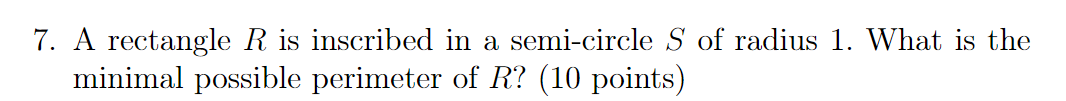### Create an Account

Home / Questions / 7. A rectangle R is inscribed in a semi-circle S of radius 1. What is the minimal possible...

# 7. A rectangle R is inscribed in a semi-circle S of radius 1. What is the minimal possible perimeter of R? (10 points)

7. A rectangle R is inscribed in a semi-circle S of radius 1. What is the minimal possible perimeter of R? (10 points)Jun 02 2021 View more View LessSubscribe To Get Solution